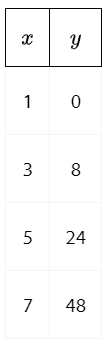# Week 5 Day 2 Opener

Below is a table of values. The two quantities, x and y, are related. What are some strategies you could use to find a relationship between x and y? Brainstorm as many ways as possible.A granola bite contains 27 calories. Most of the calories come from c grams of carbohydrates. The rest come from other ingredients. One gram of carbohydrate contains 4 calories. The equation 4c + 5 = 27 represents the relationship between these quantities. What could the 5 represent in this situation?

Priya said that neither 8 nor 3 could be the solution to the equation. Explain why she is correct.

Find the solution to the equation.

In general, what does a solution to an equation mean?